Diffraction of Particles

Diffraction of Particles

the scattering of microparticles (for example, electrons, neutrons, and atoms) by the crystals or molecules of liquids and gases, during which there arise from the initial beam of particles additional deflected beams of these particles; the direction and intensity of such deflected beams depend on the structure of the scattering object.

The diffraction of particles can be understood only on the basis of quantum theory. Diffraction is a wave phenomenon; it is observed in the propagation of a variety of waves: the diffraction of light, sound waves, waves on the surface of a liquid, and so on. From the standpoint of classical physics, diffraction is impossible in the scattering of particles. Quantum mechanics has eliminated the absolute boundary between the wave and the particle. Particle-dualism, that is, the dual nature of microparticles, is the principal proposition of quantum mechanics, which describes the behavior of microentities. Thus, the behavior of electrons in some phenomena (such as in the observation of the motion of electrons in a Wilson cloud chamber or in the measurement of electric charge in the photoelectric effect) can be described on the basis of the particle concept, while in other phenomena (especially in diffraction phenomena) it can be described only on the basis of the wave concept. The idea of “waves of matter” was advanced by the French physicist L. de Broglie in 1924 and soon received brilliant confirmation in experiments on the diffraction of particles.

According to quantum mechanics, the free motion of a particle with mass m and momentum p = mv (where v is the velocity of the particle) can be represented as a planar monochromatic wave ψ0 (a de Broglie wave) with a wavelength

(1) λ = h/p

that propagates in the same direction (for example, in the direction of the x = axis) as that in which the particle is moving (Figure 1). Here h is Planck’s constant. The relationship of ψ0 to the coordinate x is given by the formula

(2) ψ ~ cos(k0x)

where k0 = │ k0 │ = 2π/λ, the so-called wave number, while the wave vector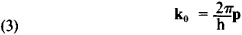is directed toward the propagation side of the wave or along the direction of motion of the particle.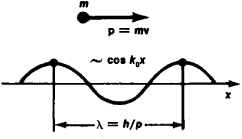Figure 1. Comparison of a wave and a freely moving particle. Shown at top is the linear motion of a particle with mass m and momentum p = mv, where v is the velocity of the particle; below, the propagation of the corresponding matter wave ψ ~ cos k0x with wavelength » = h/p.

Thus, the wave vector of a monochromatic wave connected with a freely moving microparticle is proportional to the momentum and inversely proportional to the wavelength.

Since the kinetic energy of a particle that is moving comparatively slowly is S = mv2, the wavelength can also be expressed in terms of the energy:When a particle interacts with some object, such as a crystal or molecule, its energy changes: the potential energy of this interaction is added to it, leading to a change in the particle’s motion. The character of the propagation of the wave associated with the particle changes accordingly; this takes place according to the principles common to all wave phenomena. Therefore, the main geometric principles of the diffraction of particles differ in no way from the principles governing the diffraction of any waves. A general condition for the diffraction of waves of any nature is the commensurability of the length of the incident wave λ with the distance d between the scattering centers: λ≲d.

The first experiment on the diffraction of particles, which brilliantly confirmed the original concept of quantum mechanics—that is, particle-wave dualism—was that of the American physicists K. Davisson and L. Germer (1927) on the diffraction of electrons in monocrystals of nickel (Figure 2). If electrons are accelerated by an electrical field of intensity V, then they will acquire the kinetic energy S = eV (e being the electron charge). After the numerical values are inserted in equation (4), this gives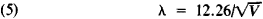Here V is expressed in volts (V) and λ is expressed in angstroms (1 υ = 10−8 cm). At voltages V on the order of 100 V (the quantity used in these experiments), so-called slow electrons with a λ on the order of 1 υ are obtained. This quantity is close to the interatomic distances d in crystals (several angstroms or less), and the ratio λ ≲ d, which is necessary for diffraction to occur, is satisfied.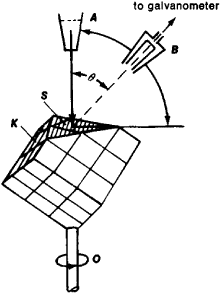Figure 2. Diagram of the Davisson-Germer experiment. (K) monocrystal of nickel, (A) electron source, (B) electron detector, (θ) angle of deflection of the electron beams. The electron beam is perpendicularly incident to the polished crystal plane S. When the crystal is rotated around the axis O, a galvanometer attached to detector B shows periodic maxima.

Crystals are highly regular. The atoms within them are arranged in a three-dimensional periodic crystal lattice, that is, they form a spatial diffraction grating for the appropriate wavelengths. The diffraction of waves in such a grating takes place as a result of scattering in systems of parallel crystal planes, on which the scattering centers are arranged in strict order. The Bragg-Vul’f condition is the necessary condition for observation of the diffraction maximum on reflection from a crystal:

(6) 2d sin θ = nλ

where θ is the angle at which the electron beam is incident to the given crystal plane (the slip angle) and d is the distance between the corresponding crystal planes.

In the Davisson and Germer experiment, maxima occurred when the electrons were “reflected” from the surface of the nickel crystal at specific angles of reflection (Figure 3). These maxima of the reflected electron beams corresponded to formula (6), and their appearance could be explained in no way other than on the basis of the concepts of waves and wave diffraction; thus, the wave properties of particles—electrons—were proved by experiment.

At higher accelerating voltages (dozens of kilovolts) electrons acquire sufficient kinetic energy to penetrate fine films of matter (with a thickness on the order of 10−5 cm, that is, thousands of υ). There then occurs the so-called transmission diffraction of fast electrons which the English scientist J. J. Thomson and the Soviet physicist P. S. Tartakovskii first investigated in polycrystalline films of aluminum and gold.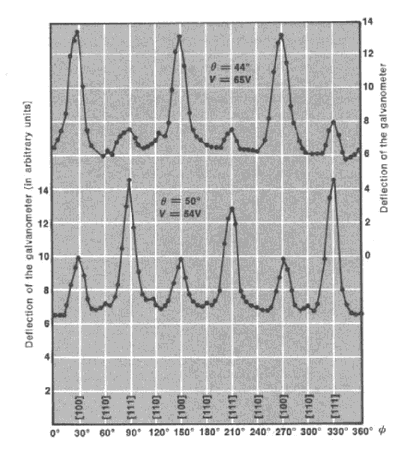Figure 3. Diffraction maxima in the Davisson-Germer experiment on electron diffraction at various angles of rotation of a crystal, φ, for two values of the angle of deflection of the electrons, θ, and two accelerating voltages V. The maxima correspond to reflection from the various crystal planes, whose indexes are indicated in brackets.

Soon afterward it also became possible to observe the diffraction phenomena of atoms and molecules. According to formula (4), the following wavelength corresponds to atoms with mass M in gaseous state in a vessel at absolute temperature T:where k is the Boltzmann constant (since the average kinetic energy of an atom g = 3/2kT). For light atoms and molecules (H, H2, He) and temperatures of hundreds of degrees Kelvin the wavelength λ is also about 1 υ. For the most part, diffracting atoms or molecules do not penetrate to the depth of the crystal; therefore, it may be assumed that their diffraction occurs through scattering from the surface of the crystal— that is, as if on a flat diffraction grating.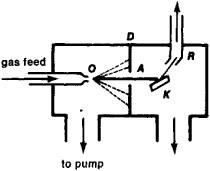Figure 4. Schematic diagram of research apparatus in the diffraction of atomic or molecular beams. (A) atomic or molecular beam, (K) crystal, (O) gas supply tube, (D) diaphragm, (R) receiver connected to a manometer. The manometer measures the pressure generated by the diffracted beam.

A molecular or atomic beam emitted from a vessel and formed by means of diaphragms is directed toward the crystal, and the “reflected” diffraction beams are in some way fixed (Figure 4). In this manner, the German scientists O. Stern and I. Estermann and other researchers in the early 1930’s observed the diffraction of atomic and molecular beams (Figure 5).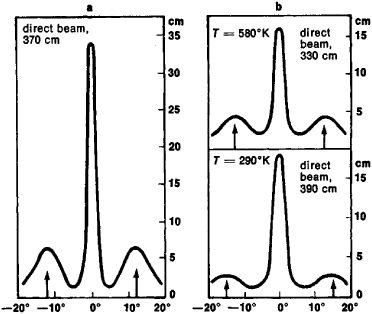Figure 5. Lithium fluoride crystal diffraction of helium atoms (a) and hydrogen molecules, at two values of absolute temperature, T (b). The angle of diffraction θ is plotted along the abscissa; the intensity of the diffracted beams (in cm of deflection of the needle of the measuring instrument), along the ordinate. In addition to a peak at θ = 0° (from the mirror reflection of the initial beam), two side peaks can be observed. At T = 580°K the side peaks are somewhat closer to the central peak than at T = 290°K. This corresponds to the decline in wavelength λ as the temperature rises; see formula (7).

The diffraction of protons and neutrons was observed later and came into wide use as a method of investigating the structure of matter.

Thus it was proven experimentally that wave properties are inherent in all microparticles, without exception.

In the broad sense of the word, diffraction scattering always occurs in the elastic scattering of various elementary particles by atoms and atomic nuclei, as well as by each other. On the other hand, the concept of the particle-wave dualism of matter was strengthened by the analysis of phenomena that had always been considered typically wave phenomena, such as the diffraction of X rays—short electromagnetic waves with a wavelength λ ≈ 0.5-5υ. At the same time, the initial and scattered X-ray beams may be considered and recorded as a flux of particles, or photons; the number of photons of X-radiation in these beams can be determined by using photon counters.

It should be emphasized that wave properties are inherent in each particle individually. This has been confirmed by an experiment conducted by V. A. Fabrikant (1947) on the diffraction of the electrons that successively pass through a sample. A standard diffraction pattern gradually arose as a certain amount of time elapsed. This meant that each of the electrons conformed to all laws of wave optics, and that the diffraction effect is a result of the interaction between the de Broglie wave of each electron and the volume of the entire crystal. The initial wave ψ0 [see formula (2)], which describes the motion of the initial electron, is converted to the scattered wave ψ on passing through the crystal.

The formation of a diffraction pattern upon the scattering of particles is interpreted in the following manner in quantum mechanics. An electron that passes through a crystal is deflected from its original motion as a result of interaction with the crystal lattice of the sample and strikes some point of a photographic plate mounted beyond the crystal in order to record electrons. Upon entering the photographic emulsion the electron is observed as a particle and induces a photochemical reaction. At first glance the incidence of the electron at a given point of the plate would seem to be totally arbitrary. But on prolonged exposure there gradually arises an ordered pattern of diffraction maxima and minima in the distribution of the electrons that have passed through the crystal.

It is impossible to predict precisely at what point of the photographic plate a given electron will strike, but the probability of its incidence at some point of the plate after scattering can be indicated. This probability is determined by the wave function of the electron, ψ, or, more accurately, by the square of its modulus (since ψ is a complex function), │ ψ2. However, when there are large numbers of trials this probability is realized as a certainty, and when an electron passes through a crystal repeatedly (or, as is the case in actual diffraction experiments, when an electron beam containing a tremendous number of particles passes through the sample) the quantity │ ψ2 now determines the intensity distribution in the diffracted beams. Thus, the resultant wave function of the electron, ψ, which can be calculated if we know ψ0 and the potential energy of interaction between the electron and the crystal, gives a complete description of the diffraction experiment in a statistical sense.

Because of the common character of the geometric principles of diffraction, the theory of the diffraction of particles borrowed a great deal from the previously developed theory of X-ray diffraction. However, the interaction of various types of particles (for example, electrons, neutrons, and atoms) with matter has a varying physical nature. Therefore, in considering the diffraction of particles in crystals, liquids, and elsewhere, it is essential to know how different particles are scattered by an isolated atom of a substance. It is precisely in the scattering of particles by individual atoms that the specifics of the diffraction of various particles become apparent. For example, the scattering of electrons is determined by the interaction of the electric charge with the electrostatic potential of the atoms, φ(r) (where r is the distance from the atom), which is the sum of the potential of the positively charged nucleus and the potential of the atom’s electron shell; the potential energy of this interaction is U = eφ(r). Neutron scattering is determined by the potential of their strong interaction with the atomic nucleus, as well as by the interaction of the magnetic moment of the neutron with the magnetic moment of the atom (the magnetic scattering of neutrons).

Quantitatively, the scattering power of an atom is characterized by a quantity called the atomic scattering amplitude, f(θ), where θ is the scattering angle and is determined by the potential energy of the interaction of particles of a given type with atoms of the scattering substance. The scattering intensity of the particles is proportional to f2(θ).

If the atomic amplitude is known and if we know the relative position of the scattering centers—the atoms of the substance in the sample (that is, if we know the structure of the scattering sample)—then we can draw up a general diffraction pattern (which is formed as a result of the interference of secondary waves originating from the scattering centers).

Theoretical calculations, confirmed by experimental measurements, show that the atomic scattering amplitude of electrons fe is maximal when θ = 0, and declines as θ increases. The quantity fe also depends on the nuclear charge (atomic number) Z and on the structure of the electron shells of the atom, increasing with Z (on the average) approximately as z for low vaiues of θ and as z for large values of θ, but exhibiting oscillations associated with the periodic character of the filling of the electron shells.

The atomic scattering amplitude of neutrons fn for thermal neutrons (neutrons with an energy of hundredths of an eV) does not depend on the scattering angle—that is, the scattering of such neutrons by the nucleus is identical in all directions (it has spherical symmetry). This is due to the fact that the atomic nucleus, with a radius on the order of 10−13 cm, is a “point” for (its size is infinitesimal in comparison to) thermal neutrons, whose wavelength is 10−8 cm. Moreover, there is no clear dependence in neutron scattering on the nuclear charge Z. Because of the presence in some nuclei of so-called resonance levels, with an energy close to the energy of thermal neutrons, the fn of such nuclei are negative.

An atom scatters electrons much more strongly than X rays and neutrons: the absolute values of the electron scattering amplitude fe are on the order 10−8 cm; for X rays, fx ~ 10−11 cm; and for neutrons, fn ~ 10−12 cm. Since the scattering intensity is proportional to the square of the scattering amplitude, electrons interact with matter (are scattered) approximately a million times more strongly than X rays (and especially than neutrons). Therefore, fine films, 10−6–10−5 cm thick, usually serve as samples for the observation of electron diffraction, while samples several mm thick must be used to observe the diffraction of X rays and neutrons.

The diffraction in any atomic system, such as a molecule or crystal, can be calculated if the coordinates of their centers r1 and the atomic amplitude fi are known for the given type of particle.

The effects of the diffraction of particles show up most clearly in crystal diffraction. However, the thermal motion of atoms in a crystal somewhat changes the conditions of diffraction, and the intensity of the diffracted beams declines as the angle θ in formula (6) increases. In the liquid diffraction of particles, or in diffraction by amorphous bodies or gas molecules, where the degree of order is far less than in crystals, several broad diffraction maxima are usually observed.

The diffraction of particles, which at one time played such an important role in establishing the dual nature of matter (particle-wave dualism) and which thereby served as the experimental substantiation of quantum mechanics, has long been one of the principal working methods in studying the structure of matter. Two important modern methods of analyzing the atomic structure of matter—electronography and neutronography—are based on the diffraction of particles.

REFERENCES

Blokhintsev, D. I. Osnovy kvantovoi mekhaniki, 4th ed. Moscow, 1963. Chapter 1, sections 7 and 8.
Pinsker, Z. G. Difraktsiia elektronov. Moscow-Leningrad, 1949.
Vainshtein, B. K. Strukturnaia elektronografiia. Moscow, 1956.
Bacon, G. Difraktsiia neitronov, Moscow, 1957. (Translated from English.)
Ramsey, N. Mokekuliarnye puchki. Moscow, 1960. (Translated from English.)

B. K. VAINSHTEIN

References in periodicals archive ?
Among his topics are the diffraction of particles in polarized crystals, positronium and muonium spin rotation and oscillations, the phenomenon of time-reversal violating magnetic field generation by a static electric field and electric field generation by a static magnetic field, spin rotation of particles moving in bent crystals and measuring the magnetic moment of short-lived particles and nuclei, and synchrotron-type radiation processes in crystals and polarization phenomena accompanying them.

Site: Follow: Share:
Open / Close